## 3.12Useful TVD schemes

For irregular 3D polyhedral meshes, only TVD schemes whose limiter function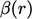avoids excessive downwind interpolation, are suﬃciently robust to be practically useful.

### Limited linear and minmod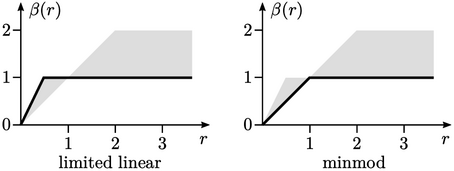The limited linear scheme — as the name suggests — maintains the linear scheme as much as possible to be TVD, before limiting to upwind to improve boundedness, according to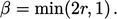(3.13)
Limited linear is a robust scheme that exhibits low diﬀusion, but has a disadvantage that it is not symmetric, according to the criterion described in Sec. 3.11 .

Like limited linear, the minmod scheme9 does not introduce any downwind interpolation. It is symmetric but is more diﬀusive by limiting more readily towards upwind, according to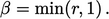(3.14)

### van Leer and van Albada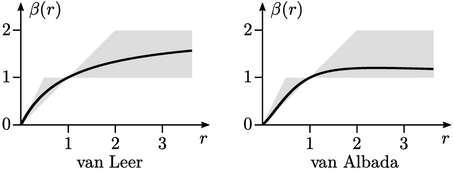There are two notable TVD schemes that: exhibit low diﬀusion; maintain symmetry through some downwind interpolation; and, have limiter functions which are continuous in gradient which avoids problems due to sudden changes in limiter values.

The van Leer scheme10 is the original limited advection scheme described by the limiter function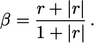(3.15)
Since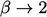as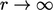, the van Leer scheme uses relatively strong downwind interpolation.

The van Albada scheme11 is described by the limiter function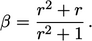(3.16)
It uses much less downwind interpolation than the van Leer scheme since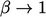as. From the diagram, it appears like a smooth-functioned, less diﬀuse version of minmod.
9Philip Roe, Characteristic-based schemes for the Euler equations, 1986.
10Bram van Leer, Towards the ultimate conservative diﬀerence scheme II. Monotonicity and conservation combined in a second order scheme, 1974.
11Gale van Albada, et al. A comparative study of computational methods in cosmic gas dynamics, 1982.

Notes on CFD: General Principles - 3.12 Useful TVD schemes